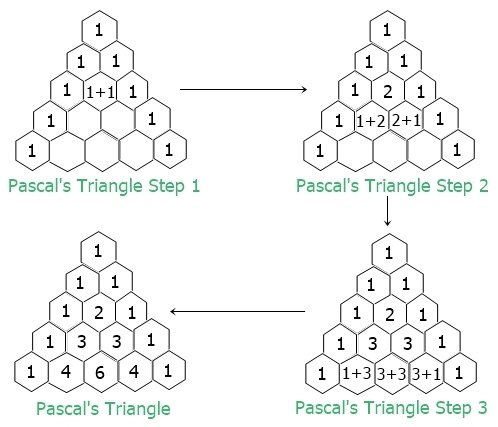# Java Program to Print Pascal Triangle

This post covers a program in Java that prints Pascal's Triangle. If you're not aware about, what Pascal's triangle is, then you can refer to Pascal's Triangle. But for now, the figure given below shows everything about Pascal's triangle:Now let's move on and create a program.

## Print Pascal's Triangle in Java

The question is, write a Java program to print Pascal's triangle. Answer to this question, is the program given below:

```public class CodesCracker
{
public static void main(String[] args)
{
int row=5, i, j, space, num;
for(i=0; i<row; i++)
{
for(space=row; space>i; space--)
{
System.out.print(" ");
}
num=1;
for(j=0; j<=i; j++)
{
System.out.print(num+ " ");
num = num*(i-j)/(j+1);
}
System.out.print("\n");
}
}
}```

The snapshot given below shows the sample output produced by above program on printing of a Pascal's triangle, of 5 lines or rows:The same program can also be created as:

```public class CodesCracker
{
public static void main(String[] args)
{
int row=5, i, j, x=1, y=0;
int[] arr = new int[row];
int[] arrTemp = new int[row];
arr = 1;
arr = 1;

for(i=0; i<row; i++)
{
for(j=(row-1); j>i; j--)
System.out.print(" ");
for(j=0; j<=i; j++)
{
if(i==0)
System.out.print("1");
else
{
if(j==0 || j==i)
System.out.print("1 ");
else
{
arrTemp[x] = arr[y] + arr[y+1];
System.out.print(arrTemp[x]+ " ");
x++;
y++;
}
}
}
System.out.print("\n");
arrTemp[x] = 1;
if(i>1)
{
y=0;
arr[y]=1;
for(x=1, y=1; y<=i; x++, y++)
arr[y] = arrTemp[x];
x=1;
y=0;
}
}
}
}```

Since the value of row is 5, in this program too, therefore you'll get the exact output as of previous program.

## Print Pascal's Triangle of Given Size in Java

This is the last program of this article, created to allow user to define the size of Pascal's triangle at run-time of the program.

```import java.util.Scanner;

public class CodesCracker
{
public static void main(String[] args)
{
int num;
Scanner s = new Scanner(System.in);

System.out.print("Enter the Row Size of Pascal Triangle: ");
int row = s.nextInt();

for(int i=0; i<row; i++)
{
for(int space=row; space>i; space--)
System.out.print(" ");
num=1;
for(int j=0; j<=i; j++)
{
System.out.print(num+ " ");
num = num*(i-j)/(j+1);
}
System.out.print("\n");
}
}
}```

Here is its sample run with user input 10 as row size of the Pascal's triangle:#### Same Program in Other Languages

Java Online Test

« Previous Program Next Program »